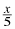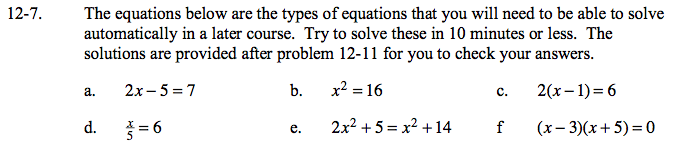### Home > GC > Chapter 12 > Lesson 12.1.1 > Problem12-7

12-7.
1. The equations below are the types of equations that you will need to be able to solve automatically in a later course. Try to solve these in 10 minutes or less. The solutions are provided after problem 12-11 for you to check your answers. Homework Help ✎

1. 2x − 5 = 7

2. x2 = 16

3. 2(x −1) = 6

4.= 6

5. 2x2 + 5 = x2 + 14

6. (x − 3)(x + 5) = 0x = 6

Remember that 16 is a perfect square, and there are two answers.

4 or −4

Distribute before simplifying.

x = 4

Multiply by the denominator.

30

Set equal to zero, then factor.

3, −3

Which numbers make this true?

3 or −5